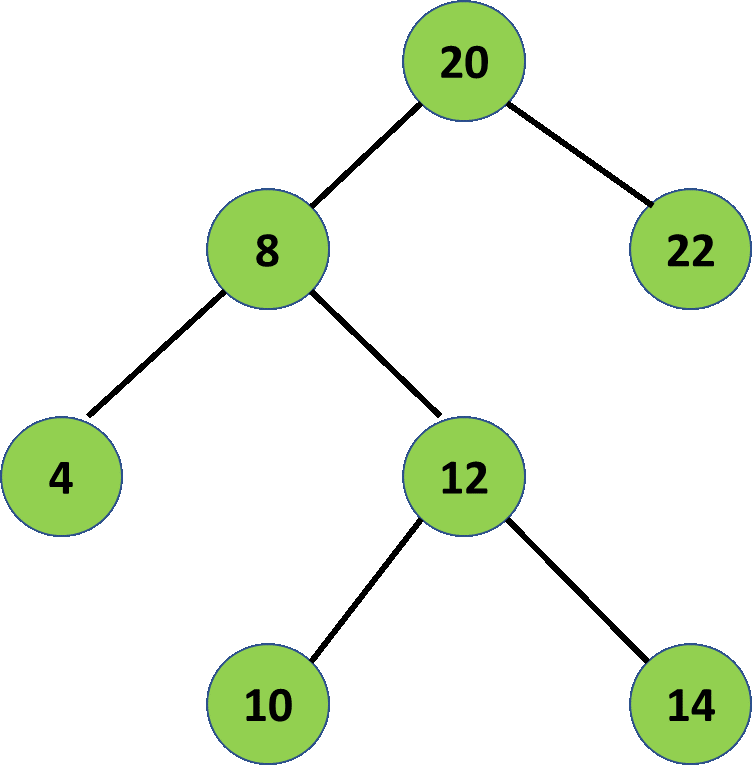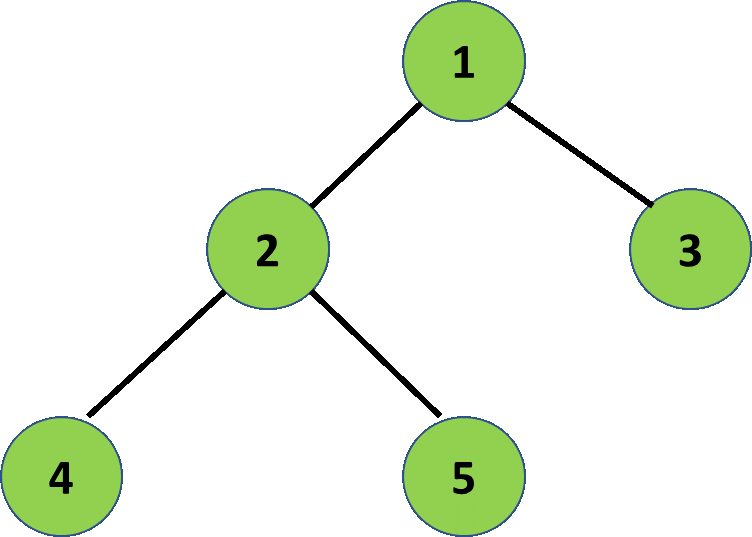# Print the nodes of Binary Tree having a grandchild

Given a Binary Tree, the task is to print the nodes that have grandchildren.

Examples:

Input:Output: 20 8
Explanation:
20 and 8 are the grandparents of 4, 12 and 10, 14.

Input:Output: 1
Explanation:
1 is the grandparent of 4, 5.

## Recommended: Please try your approach on {IDE} first, before moving on to the solution.

Approach: The idea use Recursion. Below are the steps:

1. Traverse the given tree at every node.
2. Check if each node has grandchildren node or not.
3. For any tree node(say temp) if one of the below node exists then current node is the grandparent node:
• temp->left->left.
• temp->left->right.
• temp->right->left.
• temp->right->right.
4. If any of the above exist for any node temp then the node temp is grandparent node.

Below is the implementation of the above approach:

## C++

 `// C++ program for the above approach ` `#include ` `using` `namespace` `std; ` ` `  `// A Binary Tree Node ` `struct` `node { ` `    ``struct` `node *left, *right; ` `    ``int` `key; ` `}; ` ` `  `// Function to create new tree node ` `node* newNode(``int` `key) ` `{ ` `    ``node* temp = ``new` `node; ` `    ``temp->key = key; ` `    ``temp->left = temp->right = NULL; ` `    ``return` `temp; ` `} ` ` `  `// Function to print the nodes of ` `// the Binary Tree having a grandchild ` `void` `cal(``struct` `node* root) ` `{ ` `    ``// Base case to check ` `    ``// if the tree exists ` ` `  `    ``if` `(root == NULL) ` `        ``return``; ` ` `  `    ``else` `{ ` ` `  `        ``// Check if there is a left and ` `        ``// right child of the curr node ` `        ``if` `(root->left != NULL ` `            ``&& root->right != NULL) { ` ` `  `            ``// Check for grandchildren ` `            ``if` `(root->left->left != NULL ` `                ``|| root->left->right != NULL ` `                ``|| root->right->left != NULL ` `                ``|| root->right->right != NULL) { ` ` `  `                ``// Print the node's key ` `                ``cout << root->key << ``" "``; ` `            ``} ` `        ``} ` ` `  `        ``// Check if the left child ` `        ``// of node is not null ` `        ``else` `if` `(root->left != NULL) { ` ` `  `            ``// Check for grandchildren ` `            ``if` `(root->left->left != NULL ` `                ``|| root->left->right != NULL) { ` `                ``cout << root->key << ``" "``; ` `            ``} ` `        ``} ` ` `  `        ``// Check if the right child ` `        ``// of node is not null ` `        ``else` `if` `(root->right != NULL) { ` ` `  `            ``// Check for grandchildren ` `            ``if` `(root->right->left != NULL ` `                ``|| root->right->right != NULL) { ` `                ``cout << root->key << ``" "``; ` `            ``} ` `        ``} ` ` `  `        ``// Recursive call on left and ` `        ``// right subtree ` `        ``cal(root->left); ` `        ``cal(root->right); ` `    ``} ` `} ` ` `  `// Driver Code ` `int` `main() ` `{ ` `    ``// Given Tree ` `    ``struct` `node* root = newNode(1); ` `    ``root->left = newNode(2); ` `    ``root->right = newNode(3); ` `    ``root->left->left = newNode(4); ` `    ``root->left->right = newNode(5); ` ` `  `    ``// Function Call ` `    ``cal(root); ` `    ``return` `0; ` `} `

Output:

```1
```

Time Complexity: O(N), where N is the number of nodes.
Auxiliary Space: O(1)My Personal Notes arrow_drop_upCheck out this Author's contributed articles.

If you like GeeksforGeeks and would like to contribute, you can also write an article using contribute.geeksforgeeks.org or mail your article to contribute@geeksforgeeks.org. See your article appearing on the GeeksforGeeks main page and help other Geeks.

Please Improve this article if you find anything incorrect by clicking on the "Improve Article" button below.